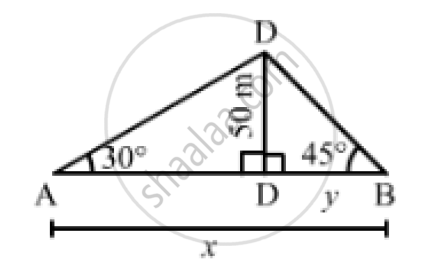# Two Men Are on Opposite Side of Tower. They Measure Angles of Elevation of the Top of Tower as 30 and 45 Respectively. If the Height of the Tower is 50 Meters, Find the Distance Between the Two Men. - Mathematics

Two men are on opposite side of tower. They measure the angles of elevation of the top of the tower as 30 and 45 respectively. If the height of the tower is 50 meters, find the distance between the two men.

#### Solution

Let CD be the tower and A and B be the positions of the two men standing on the opposite sides.
Thus, we have:
∠DAC = 30°,  ∠DBC = 45° and CD = 50 m
Let AB =  xmand BC =  ymsuch that AC = (x - y)m.In the right ΔDBC,we have:

(CD)/(BC) = tan 45° = 1

⇒ 50/y = 1

⇒  y = 50 m

In the right  ΔACD,we have:

(CD)/(AC) = tan 30° = 1/ sqrt(3)

⇒  50/ ((x-y)) = 1/ sqrt(3)

x -y = 50 sqrt(3)

On putting y  = 50in the above equation, we get:

x -50= 50sqrt(3)

⇒  x = 50 + 50 sqrt(3) = 50 ( sqrt(3) +1) = 136.6m

∴Distance between the two men = AB = x = 136.6m

Concept: Heights and Distances
Is there an error in this question or solution?
Chapter 14: Height and Distance - Exercises

#### APPEARS IN

RS Aggarwal Secondary School Class 10 Maths
Chapter 14 Height and Distance
Exercises | Q 12

Share